# Checking for the Presence of Specified Values Within a Range in Microsoft Excel

To check for the presence of specified values within a range, we can use the AND function along with the COUNTIF function in Microsoft Excel.

COUNTIF: This function is used to count the number of cells within a range which meet a given condition.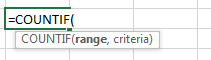For Example:-We have a data table in range A2:A11.  We need to find out how many times the states are repeating in this range.

• Select the cell B2, and write the formula =Countif(A2:A11, A2) and press the Enter key on the keyboard.
• The Countiffunction will return 4which means that “Washington” is repeating 4 times in column A.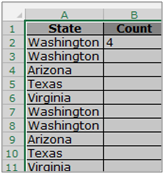AND:- This function is used to check whether all arguments are true and returns TRUE if all arguments are TRUE. If one argument is false, FALSE is returned.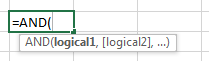For example:Cell A1 contains student name, B1 contains 50, and we need to check if the value in cell B1 is more than 40 as well as less than 60.

• Write the formula in cell C1
• =AND(B2>40,B2<60), press Enter on the keyboard.
• The function will return True as the number 50 is greater than 40 as well as less than 60.
• If we will change the number to a number which is less than 40 and greater than 60, the function will return False, as in the example below.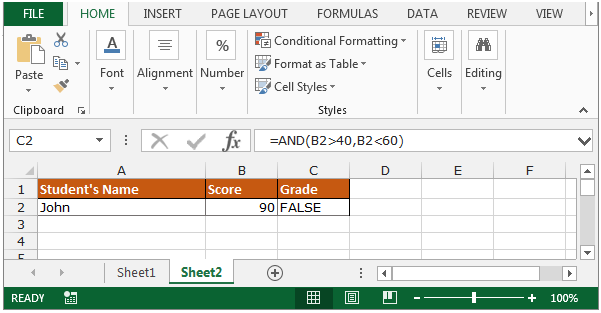Lets use the COUNTIF function to check the presence of specified values within the range and the AND function will assist us too.

We have data for certain items in range A2:C6 and the 2ndlist is in Column E.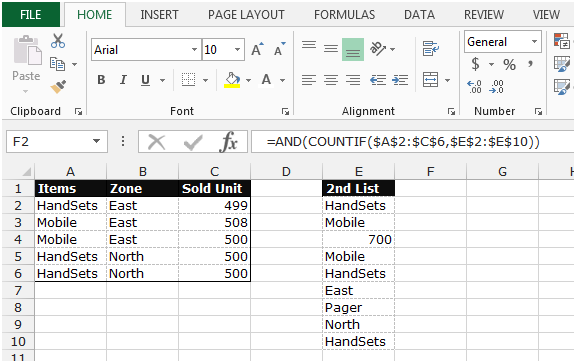We need to check the presence of the values in column E in the columns A:C.

• Select the cell F2.
• Write the formula=AND(COUNTIF(\$A\$2:\$C\$6,\$E\$2:\$E\$10))
• Press Ctrl+Shift + Enteron the keyboard
• The function will return TRUE which means that the value in cell E2 is present in the range A2:C6.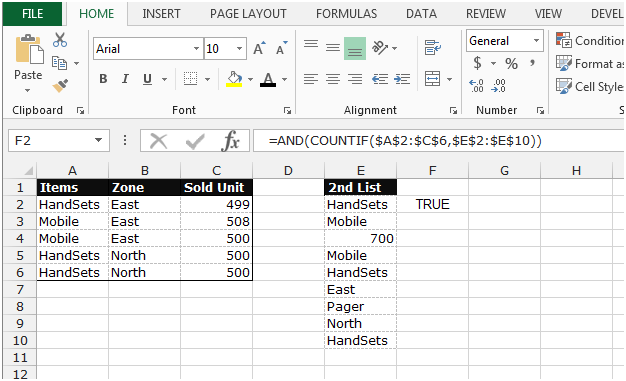• Copy the same formula by pressing the key Ctrl+C and paste in the range F3:F10 by pressing the key Ctrl+V on your keyboard.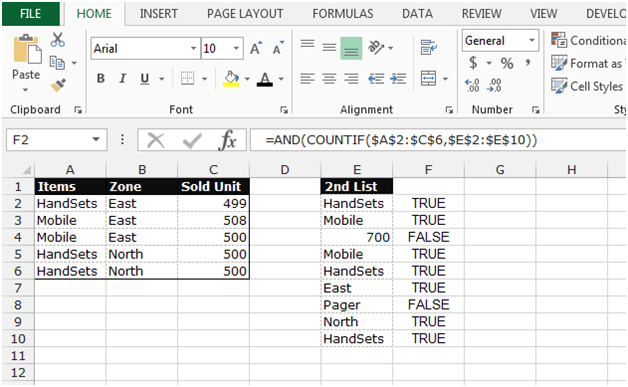Note: This is an array function, hence you cannot paste the formula in multiple cells. You will have to paste the formula one by one in each cell.

After pasting the formula in each cell, we get 2 cells with False and the remaining True. 700 has a False value in F4 as 700 does not exist in column C. Pager is also False in cell F8 as Pager does not exist in colum A.

This is the way we can check the presence of the specified values within a range.